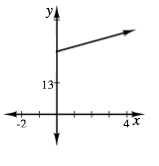### Home > INT3 > Chapter 1 > Lesson 1.2.1 > Problem1-70

1-70.

Carlo got a pet snake as a birthday present. On his birthday, the baby snake was just $26$ cm long. He has been measuring its length weekly and has noticed that it has been growing approximately $2$ cm each week.

1. Create multiple representations (table, graph, and equation) of the situation for which the input is time in weeks since Carlo’s birthday, and the output is the length of the snake in centimeters.

Refer to problem 1-39.

2. If the snake continues to grow at the same rate, when will it be $1$ meter ($100$ cm) long? How can you see this in each representation?

What point represents the length of $100$ cm in each representation?
Show how to find the point using your graph, table, and equation.Create a representation by completing the table in the eTool below.
Click the link at right for the full version of the eTool: Int3 1-70 HW eTool